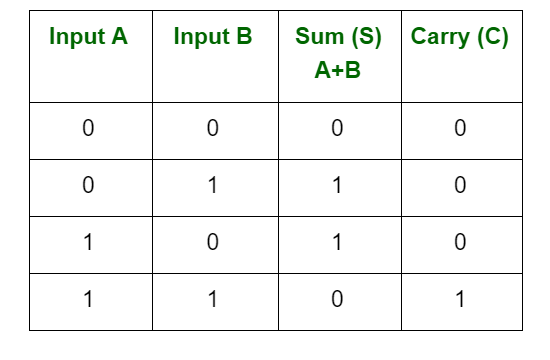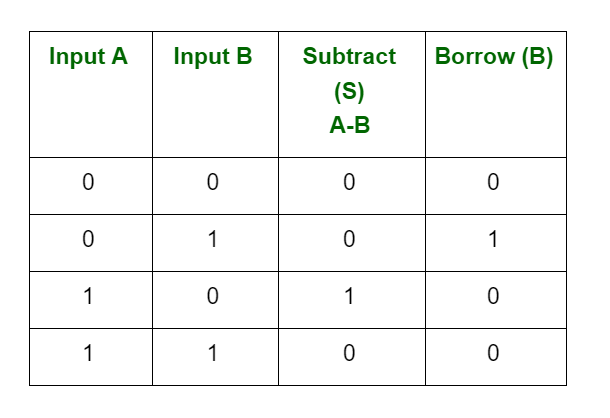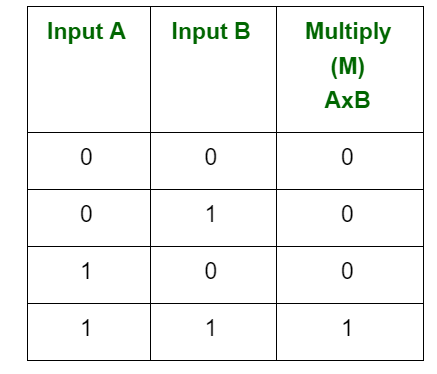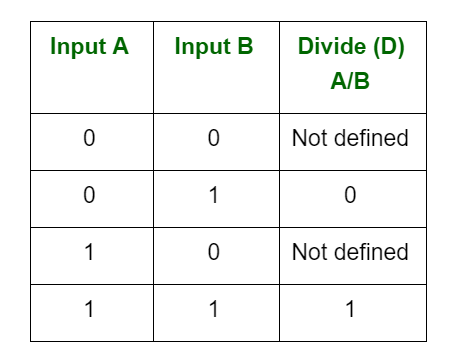# Arithmetic Operations of Binary Numbers

• Last Updated : 31 Jul, 2021

Binary is a base-2 number system that uses two states 0 and 1 to represent a number. We can also call it to be a true state and a false state. A binary number is built the same way as we build the normal decimal number

Binary arithmetic is an essential part of various digital systems. You can add, subtract, multiply, and divide binary numbers using various methods. These operations are much easier than decimal number arithmetic operations because the binary system has only two digits: 0 and 1.

Attention reader! Don’t stop learning now.  Practice GATE exam well before the actual exam with the subject-wise and overall quizzes available in GATE Test Series Course.

Learn all GATE CS concepts with Free Live Classes on our youtube channel.

Binary additions and subtractions are performed as same in decimal additions and subtractions. When we perform binary additions, there will be two outputs: Sum (S) and Carry (C).

1. There are four rules for binary addition:2. There are four rules for binary subtraction:Here 0-1 should be 1 and 1

Borrow 1 is required from the next higher order bit to subtract 1 from 0. So, the result became 0.

3. There are four rules for binary multiplication:Multiplication is always 0, whenever at least one input is 0.

4. There are four parts in any division: Dividend, Divisor, quotient, and remainder.The result is always not defined, whenever the divisor is 0.

My Personal Notes arrow_drop_up